# Normal Equation

For an $m \times n$ matrix $A$ and a vector $b \in \mathbb{R}^m$, the linear system $Ax = b$ is to find the optimal solution $x \in \mathbb{R}^n$.

## 1. The Residual Minimization

The problem $Ax = b$ can be replaced by the problem minimizing the residual, $\left\| b - Ax \right\|_2^2$. Let $A_i$ be the $i$-th row vector of $A$. Then the cost function $f(x)$ can be defined as follows: \begin{aligned} f(x) &= \left\| b - Ax \right\|_2^2 = \left\| \left(\begin{array}{c} b_1 - A^t_1 x \\ \vdots \\ b_m - A^t_m x \end{array}\right) \right\|_2^2 = \sum_{k} (b_k - A^t_k x)^2 \end{aligned}

For minimizing $f$, finding the critical point is required. Let $a_{ij}$ be the $(i, j)$ element of $A$, then \begin{aligned} \frac{\partial f}{\partial x_i} &= \sum_{k} 2(b_k - A^t_k x) \frac{\partial}{\partial x_i} (b_k - A^t_k x) = 2 \sum_{k} (b_k - A^t_k x) (- a_{ki}) = 0 \\ &\implies \sum_{k} a_{ki} A^t_k x = \sum_{k} a_{ki} b_k = \left(\begin{array}{ccc} a_{1i} & \cdots & a_{mi} \end{array}\right) \left(\begin{array}{c} A^t_1 \\ \vdots \\ A^t_m \end{array}\right) x = \left(\begin{array}{ccc} a_{1i} & \cdots & a_{mi} \end{array}\right) \left(\begin{array}{c} b_1 \\ \vdots \\ b_m \end{array}\right) \\ &\implies \left(\begin{array}{ccc} a_{11} & \cdots & a_{m1} \\ & \vdots & \\ a_{1n} & \cdots & a_{mn} \end{array}\right) \left(\begin{array}{c} A^t_1 \\ \vdots \\ A^t_m \end{array}\right) x = \left(\begin{array}{ccc} a_{11} & \cdots & a_{m1} \\ & \vdots & \\ a_{1n} & \cdots & a_{mn} \end{array}\right) \left(\begin{array}{c} b_1 \\ \vdots \\ b_m \end{array}\right) \\ \\ &\implies \color{red}{A^tAx = A^t b} \end{aligned}

This equation is called normal equation. Moreover, $A^tA$ is positive (semi-)definite because $x^t A^t Ax = (Ax)^t (Ax) = \left\| Ax \right\|_2^2 \ge 0$, so it minimizes $f$. Especially, if $\left\| Ax \right\|_2^2 \not = 0$, it tells that there is no $x$ such that $Ax = 0$ except $x = 0$. It also means that $A$ is nonsingular whose column vectors are linearly independent. Therefore, the solution of $A^t Ax = A^t b$ is the optimal solution of $Ax = b$ when $A$ is nonsingular.

## 2. Another Approach When $m > n$

In this case, $A$ maps the lower dimension to the higher dimension. For example, when $m = 3$ and $n = 2$, there may be a point $b$ in the objective space which $Ax$ cannot cover.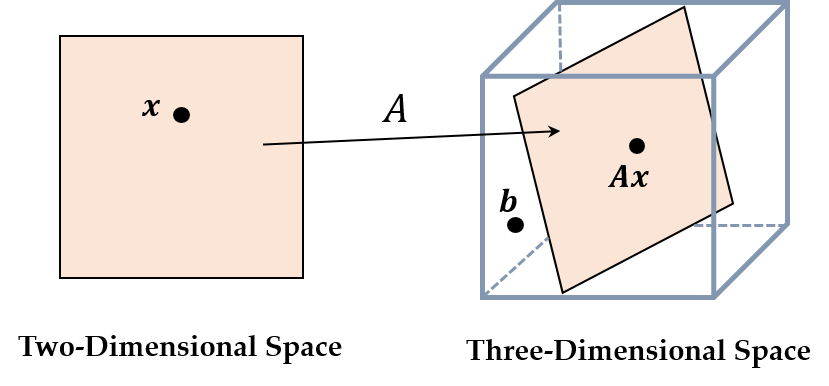So, the linear system $Ax = b$ cannot be solved exactly. However, we can still find the point which is closest to $b$. Let $y = Ax$ be the closest point to $b$.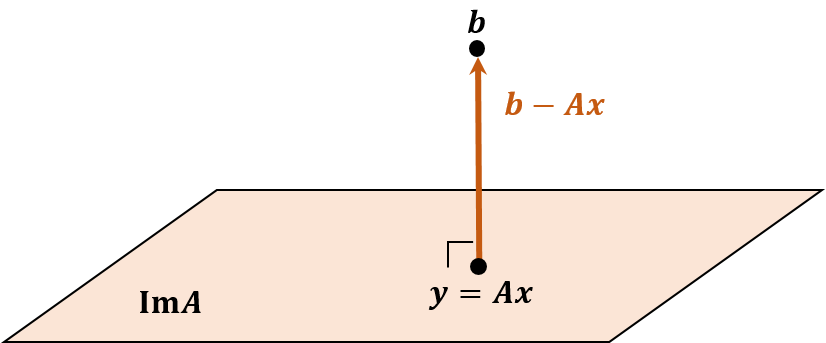When $y$ is closest to $b$, the vector $b - y = b - Ax$ is perpendicular to the normal of hyperplane $Im A$. Suppose that $e_1, \cdots, e_n$ are the standard basis in the original space. Then $Ae_1, \cdots, Ae_n$ consist of the basis of the objective space. In other words, each column vector of $A$ consists of the hyperplane $Im A$, which means it is perpendicular to $b - Ax$. Therefore, $A^t (b - Ax) = 0$, it yields the normal equation. Accordingly, the normal equation estimates the optimal solution of $Ax = b$ even though the exact solution cannot be found.

## 3. Another Approach Using Projector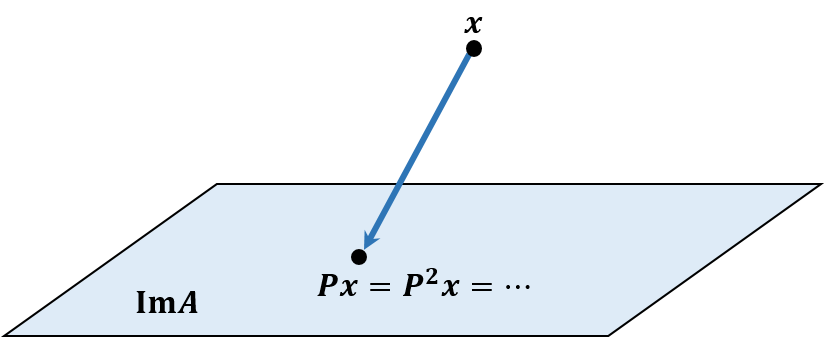A square matrix $P$ is idempotent if $P^2 = P$, and it is called projector. $P$ projects any vector to $Im P$ and if the vectors are already on $Im P$, they would be still after projected. If $P$ is symmetric, it is called orthogonal projector, and $I - P$ is also orthogonal projector to the hyperplane which is perpendicular to $Im P$. Of course, $(I - P)^2 = I - 2P + P^2 = I - P$. For a vector $v$, \begin{aligned} (Pv)^t ((I - P)v) &= v^t P^t (v - Pv) = v^t P (v - Pv) \\ &= v^t P v - v^t P^2 v = v^t P v - v^t P v = 0 \\ \\ &\implies Pv \perp (I - P)v \\ &\implies v = Pv + (I - P)v \end{aligned}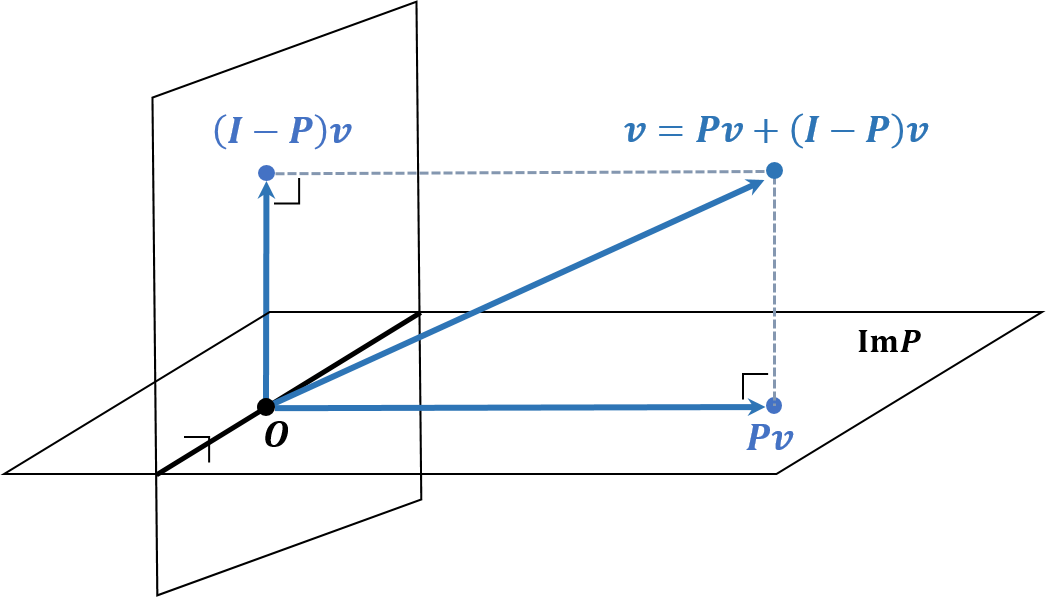Now, to apply this to solving $Ax = b$, assume that $P$ is the orthogonal projector onto $span A$, which means $PA = A$. Then the cost function $f(x)$ can be rewritten as \begin{aligned} f(x) = \left\| b - Ax \right\|^2_2 &= \left\| P(b - Ax) + (I - P)(b - Ax) \right\|^2_2 \\ &= \left\| P(b - Ax) \right\|^2_2 + \left\| (I - P)(b - Ax) \right\|^2_2 \\ &= \left\| Pb - PAx \right\|^2_2 + \left\| (I - P)b - (I - P)Ax \right\|^2_2 \\ &= \left\| Pb - Ax \right\|^2_2 + \left\| (I - P)b \right\|^2_2 \end{aligned}

Therefore, for minimizing this cost function, $\left\| Pb - Ax \right\|^2_2$ should be zero. \begin{aligned} Pb - Ax &= A^t Pb - A^t Ax = A^t P^t b - A^t Ax \\ &= (PA)^t b - A^t Ax = A^t b - A^t Ax = O \end{aligned}

Note that it produces the normal equation as well.

## 4. Evaluation

The solution from the normal equation can be evaluated by the condition number and angle between $b$ and $Ax$. The condition number of $A$ tells how close to singular $A$ is. However, if $m \not = n$, $A$ is not invertible, so the pseudoinverse $A^{+} = (A^t A)^{-1} A^t$ should be introduced. In this case, the condition number of $A$ is \begin{aligned} Cond_2 (A) = \left\| A \right\|_2 \left\| A^{+} \right\|_2 \end{aligned}

Meanwhile, as the angle between $b$ and $Ax$ is smaller, $b$ is closer to $Ax$, so it is a good measure to find whether the solution is well-conditioned. This angle can be obtained as $\cos \theta = \frac{\left\| Ax \right\|_2}{\left\| b \right\|_2}$.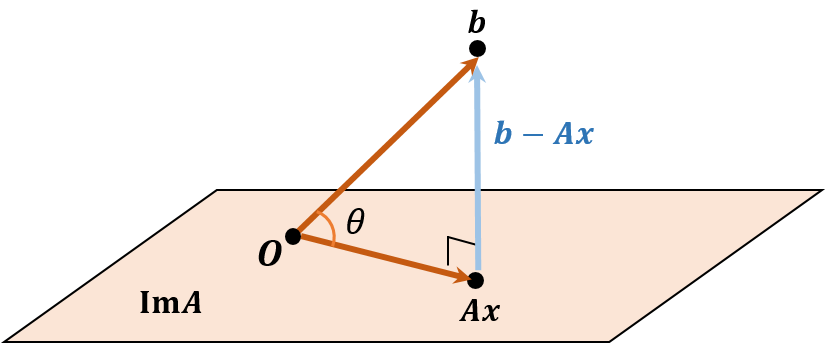## Reference

 Michael T. Heath, Scientific Computing: An Introductory Survey. 2nd Edition, McGraw-Hill Higher Education.